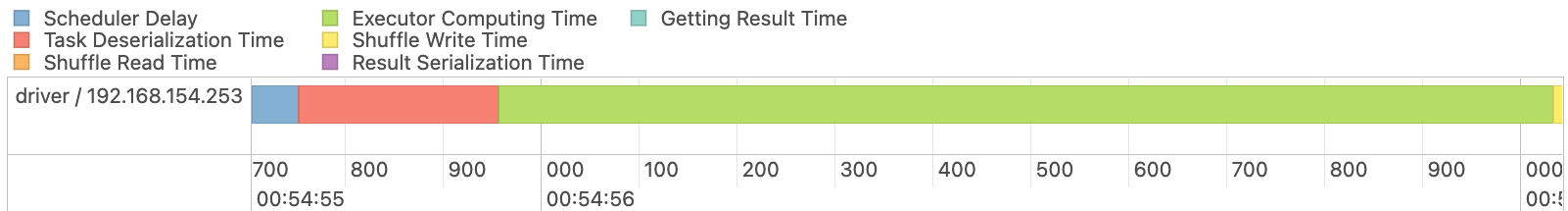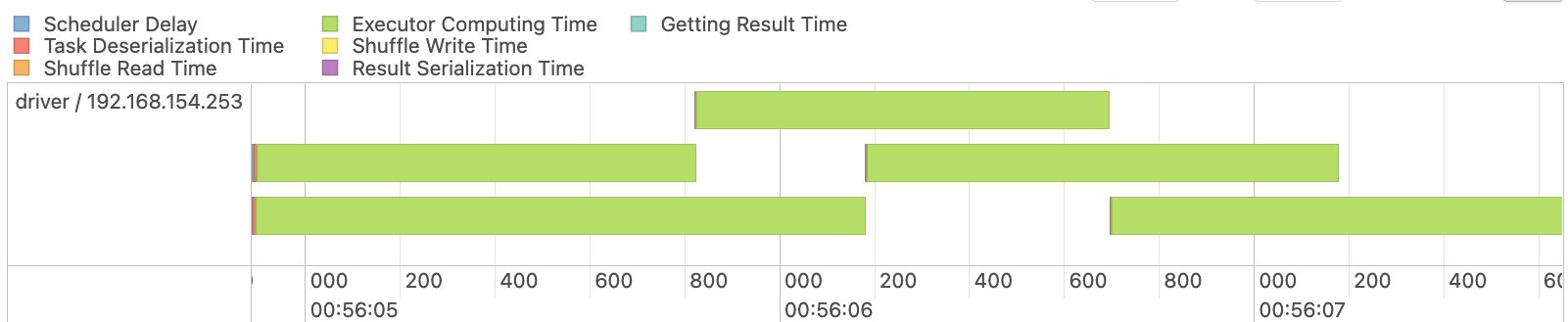# Distributed database access with Spark and JDBCBy default, when using a JDBC driver (e.g. Postgresql JDBC driver) to read data from a database into Spark only one partition will be used.

So if you load your table as follows, then Spark will load the entire table test_table into one partition

val df = spark.read
.format("jdbc")
.option("url", "jdbc:postgresql://localhost:5432/testdb")
.option("driver", "org.postgresql.Driver")
.option("dbtable", "test_table")


You can confirm this by checking the Spark UI and you will notice that the load job had only one task as you can see in the following screenshot.## Partitioning on numeric or date or timestamp columns

Luckily, Spark provides few parameters that can be used to control how the table will be partitioned and how many tasks Spark will create to read the entire table.

You can check all the options Spark provide for while using JDBC drivers in the documentation page - link. The options specific to partitioning are as follows:

Option Description
partitionColumn The column used for partitioning, it has to be numeric or date or timestamp column.
lowerBound The minimum value in the partition column
upperBound The maximum value in the partition column
numPartitions The maximum number of partitions that can be used for parallel processing in table reading and writing. This also determines the maximum number of concurrent JDBC connections.

Note if the parition column is numeric then the values of lowerBound and upperBound has to be covertable to long or spark will through a NumberFormatException.

### Using a table for partitioning

Now, when using those options and having a dbtable option set, the logic to read a table with Spark become something like this

val df = spark.read
.format("jdbc")
.option("url", "jdbc:postgresql://localhost:5432/testdb")
.option("driver", "org.postgresql.Driver")
.option("dbtable", "test_table")
.option("partitionColumn", "test_column")
.option("numPartitions", "10")
.option("lowerBound", "0")
.option("upperBound", "100")


As you can imagine this approach will provide much more scalability then the earlier read option. You can confirm this by looking in the Spark UI and see that spark created numPartitions partitions and that each one of them has more or less (upperBound - lowerBound) / numPartitions rows. The following screenshot is a screenshot that shows how spark partitioned the red job.### Using a query for partitioning

We can also use a query instead of a table for partitioing, this is actually strightforward as we just need to convert the query (e.g. select a, b, from table) to something like (select a, b, from table) as subquery then use it in the dbtable option.

val df = spark.read
.format("jdbc")
.option("url", "jdbc:postgresql://localhost:5432/testdb")
.option("driver", "org.postgresql.Driver")
.option("dbtable", "(select a, b, from table) as subquery")
.option("partitionColumn", "test_column")
.option("numPartitions", "10")
.option("lowerBound", "0")
.option("upperBound", "100")


### How to get the boundaries

Getting the values for lowerBound and upperBound should be straightforward, either set them to specific values or use actual min and max values in the table with a query like this:

val url = "jdbc:postgresql://localhost:5432/testdb"
val connection = DriverManager.getConnection(url)
val stmt = connection.createStatement()
val query = s"select count($partitionColumn) as count_value, min($partitionColumn) as min_value, max($partitionColumn) as max_value from$table"
val resultSet = stmt.executeQuery(query)

var rows = ListBuffer[Map[String, String]]()
while (resultSet.next()) {
rows += columns.map(column => (column, resultSet.getString(column))).toMap
}
val values = rows.toList
val lowerBound = values(0)("min_value")
val upperBound = values(0)("max_value")


On the other hand, setting an appropriate value for numPartitions is not that straightforward and you need to know in front how big is the table and have an estimate on how do you spread the data over multiple partitions in Spark.

## Partitioning on string columns

Unfortunately, the previous partitioning support that Spark provides out of the box does not work with columns of type string.

One way to address this is to calculate the integer division of the hash value of the column over the number of partitions and pass this in a where, this will assign each row to a partition identified as partitionId. The SQL query would look like this

select * from test_table where hash(partitionColumn) % numPartitions = partitionId


We can easily do this with one of the overloaded of the jdbc API in Spark’s DataFrameReader that accepts an array of SQL where clauses. We just need to create one where clause for each partition and use the hashing trick as follows:

val predicateFct = (partition: Int) => s"""hash("$partitionColumn") %$numPartitions = $partition""" val predicates = (0 until numPartitions).map{partition => predicateFct(partition)}.toArray  Then we can simply use those predicates to create partitions when Spark loads the table as follows: val df = spark.read .format("jdbc") .option("driver", "org.postgresql.Driver") .option("dbtable", "test_table") .jdbc(url, "test_table", predicates, jdbcProperties)  Putting everything together, the logic for partitioning on string columns can be achieved with the following snippet: val numPartitions = 10 val partitionColumn = "partitionColumn" // Define JDBC properties val url = "jdbc:postgresql://localhost:5432/testdb" val jdbcProperties = new java.util.Properties() properties.put("url", url) properties.put("user", "username") properties.put("password", "password") // Define the where clauses to assign each row to a partition val predicateFct = (partition: Int) => s"""hash("$partitionColumn") % $numPartitions =$partition"""
val predicates = (0 until numPartitions).map{partition => predicateFct(partition)}.toArray

// Load the table into Spark

Note: You need to make sure the database you’re trying to read from support hash functions. In fact, the support for hashing may differt from a database to another. For instance MySQL support hashing functions like md5 other databases may not.